# 2006COMC加拿大数学公开赛真题免费下载

### 真题与答案下载### 2006 COMC真题免费下载

Part A共8题，每题5分

Part B共4题，每题10分

#### 部分真题预览：

Part A :

3)In ΔABC, M is the midpoint of BC, as shown. If ∠ABM = 15° and ∠AMC = 30°, what is the size of ∠BCA?6)Determine the number of integers n that satisfy all three of the conditions below:

• each digit of n is either 1 or 0,
• n is divisible by 6, and
• 0 < n < 107.

8)What is the probability that 2 or more successive heads will occur at least once in 10 tosses of a fair coin?

Part B :

1)Piotr places numbers on a 3 by 3 grid using the following rule, called “Piotr’s Principle”:
For any three adjacent numbers in a horizontal, vertical or diagonal line, the middle number is always the average (mean) of its two neighbours.

1. Using Piotr’s principle, determine the missing numbers in the grid to the right. (You should fill in the missing numbers in the grid in your answer booklet.)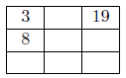2. Determine, with justification, the total of the nine numbers when the grid to the right is completed using Piotr’s Principle.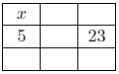3. Determine, with justification, the values of x and y when the grid to the right is completed using Piotr’s Principle.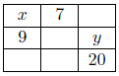3)

1. In the diagram, trapezoid ABCD has parallel sides AB and DC of lengths 10 and 20, respectively. Also, the length of AD is 6 and the length of BC is 8. Determine the area of trapezoid ABCD.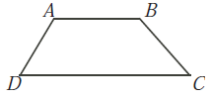2. In the diagram, PQRS is a rectangle and T is the midpoint of RS. The inscribed circles of 4PTS and 4RTQ each have radius 3. The inscribed circle of 4QPT has radius 4. Determine the dimensions of rectangle PQRS.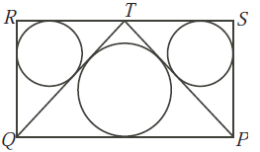### 2006 COMC加拿大数学奥赛完整版答案免费下载